# Dynamic Programming : Frog Jump (DP 3)

Problem Statement:

Given a number of stairs and a frog, the frog wants to climb from the 0th stair to the (N-1)th stair. At a time the frog can climb either one or two steps. A height[N] array is also given. Whenever the frog jumps from a stair i to stair j, the energy consumed in the jump is abs(height[i]- height[j]), where abs() means the absolute difference. We need to return the minimum energy that can be used by the frog to jump from stair 0 to stair N-1.

```Examples: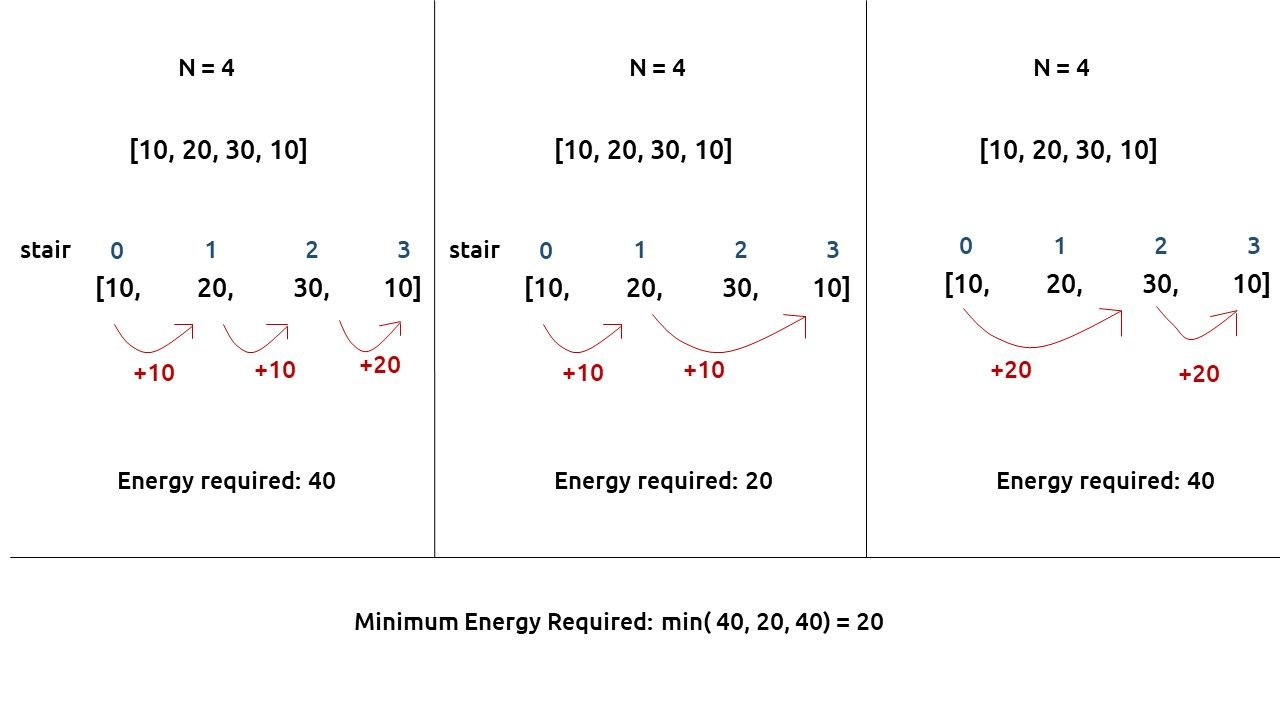```

Pre-req: Recursion, Dynamic Programming Introduction

### Solution :

As the problem statement states to find the minimum energy required, two approaches should come to our mind, greedy and dynamic programming.

First, we will see why a greedy approach will not work?

The total energy required by the frog depends upon the path taken by the frog. If the frog just takes the cheapest path in every stage it can happen that it eventually takes a costlier path after a certain number of jumps. The following example will help to understand this.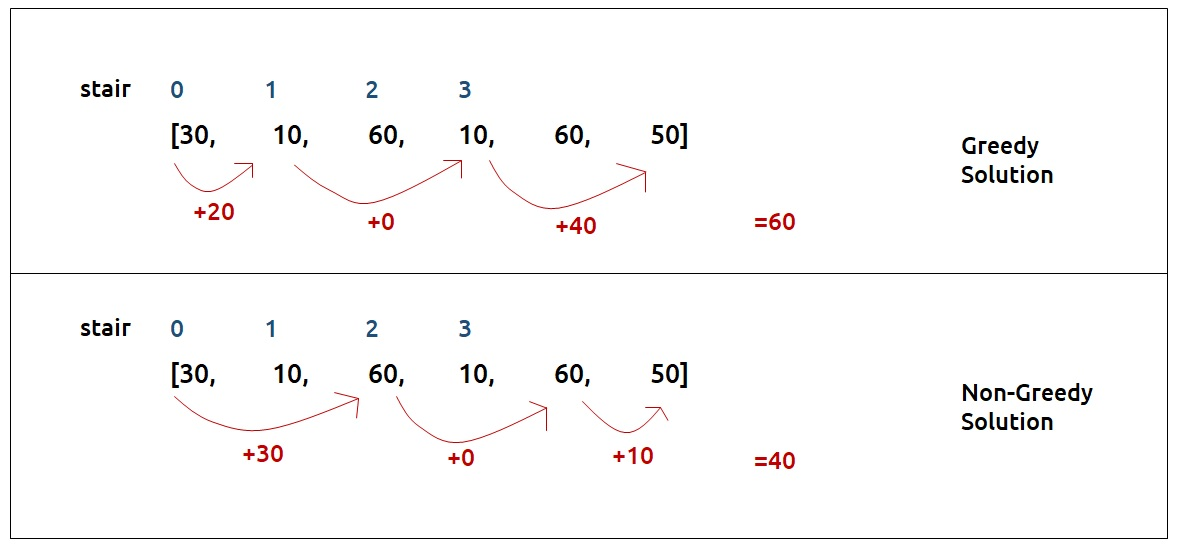Therefore a greedy solution will not work and we need to try all possible paths to find the answer.

Steps to form the recursive solution

We will recap the steps discussed in the previous article to form the recursive solution.

Step 1: Express the problem in terms of indexes

• This can be easily done as there are array indexes [0,1,2,…, n-1].
• We can say that f(n-1) signifies the minimum amount of energy required to move from stair 0 to stair n-1.
• Therefore f(0) simply should give us the answer as 0(base case).

Step 2: Try all the choices to reach the goal.

The frog can jump either by one step or by two steps. We will calculate the cost of the jump from the height array. The rest of the cost will be returned by the recursive calls that we make

Our pseudocode till this step will be: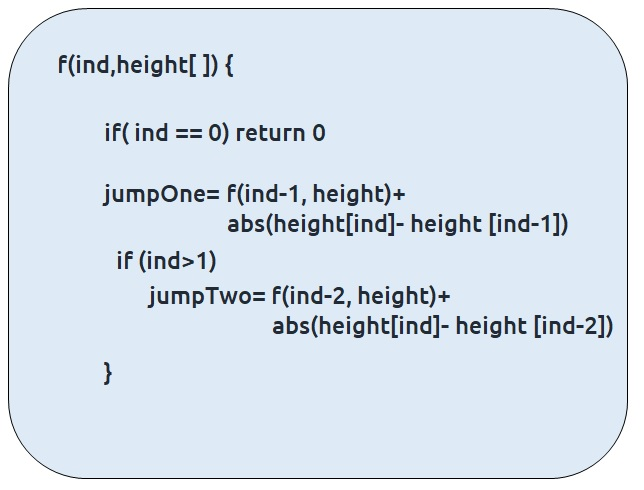Step 3: Take the minimum of all the choices

As the problem statement asks to find the minimum total energy, we will return the minimum of two choices of step2.

Also at ind=1, we can’t try the second choice so we will only make one recursive call.

The base case will be when we want to go to the 0th stair, then we have only one option.

Our final pseudo-code will be: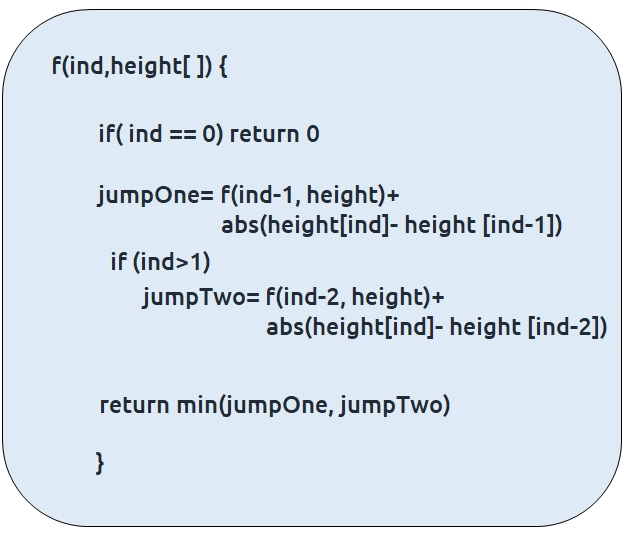Once we form the recursive solution, we can use the approach told in Dynamic Programming Introduction to convert it into a dynamic programming one.

Memoization approach

Steps to convert Recursive code to memoization solution:

• Create a dp[n] array initialized to -1.
• Whenever we want to find the answer of a particular value (say n), we first check whether the answer is already calculated using the dp array(i.e dp[n] != -1 ). If yes, simply return the value from the dp array.
• If not, then we are finding the answer for the given value for the first time, we will use the recursive relation as usual but before returning from the function, we will set dp[n] to the solution we get.

Recursion tree diagram: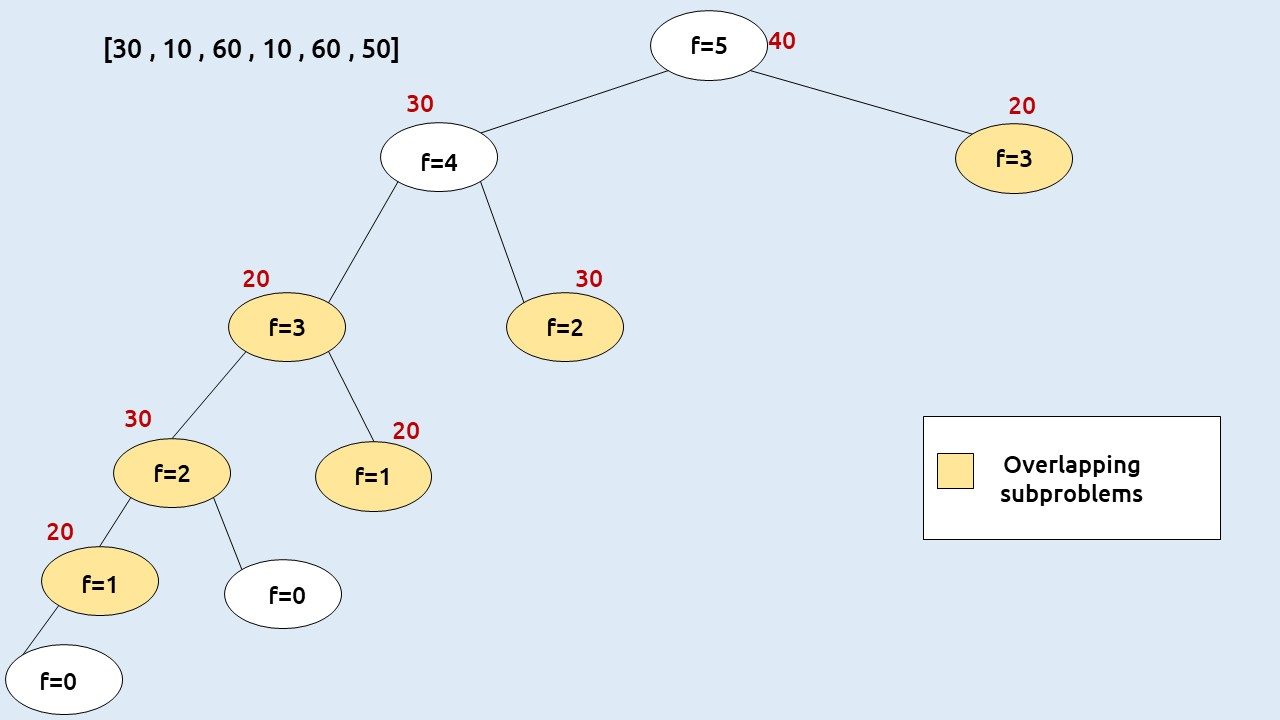Note: To watch a detailed dry run of this approach, please watch the video attached below

Code:

## C++ Code

``````#include <bits/stdc++.h>

using namespace std;

int solve(int ind, vector<int>& height, vector<int>& dp){
if(ind==0) return 0;
if(dp[ind]!=-1) return dp[ind];
int jumpTwo = INT_MAX;
int jumpOne= solve(ind-1, height,dp)+ abs(height[ind]-height[ind-1]);
if(ind>1)
jumpTwo = solve(ind-2, height,dp)+ abs(height[ind]-height[ind-2]);

return dp[ind]=min(jumpOne, jumpTwo);
}

int main() {

vector<int> height{30,10,60 , 10 , 60 , 50};
int n=height.size();
vector<int> dp(n,-1);
cout<<solve(n-1,height,dp);
}
``````

Output: 40

Time Complexity: O(N)

Reason: The overlapping subproblems will return the answer in constant time O(1). Therefore the total number of new subproblems we solve is ‘n’. Hence total time complexity is O(N).

Space Complexity: O(N)

Reason: We are using a recursion stack space(O(N)) and an array (again O(N)). Therefore total space complexity will be O(N) + O(N) ≈ O(N)

## Java Code

``````import java.util.*;
class TUF{
static int solve(int ind,int[] height,int[] dp){
if(ind==0) return 0;
if(dp[ind]!=-1) return dp[ind];
int jumpTwo = Integer.MAX_VALUE;
int jumpOne= solve(ind-1, height,dp)+ Math.abs(height[ind]-height[ind-1]);
if(ind>1)
jumpTwo = solve(ind-2, height,dp)+ Math.abs(height[ind]-height[ind-2]);

return dp[ind]=Math.min(jumpOne, jumpTwo);
}

public static void main(String args[]) {

int height[]={30,10,60 , 10 , 60 , 50};
int n=height.length;
int dp[]=new int[n];
Arrays.fill(dp,-1);
System.out.println(solve(n-1,height,dp));
}
}``````

Output: 40

Time Complexity: O(N)

Reason: The overlapping subproblems will return the answer in constant time O(1). Therefore the total number of new subproblems we solve is ‘n’. Hence total time complexity is O(N).

Space Complexity: O(N)

Reason: We are using a recursion stack space(O(N)) and an array (again O(N)). Therefore total space complexity will be O(N) + O(N) ≈ O(N)

Tabulation approach

• Declare a dp[] array of size n.
• First initialize the base condition values, i.e dp as 0.
• Set an iterative loop which traverses the array( from index 1 to n-1) and for every index calculate jumpOne and jumpTwo and set dp[i] = min(jumpOne, jumpTwo).

Code:

## C++ Code

``````#include <bits/stdc++.h>

using namespace std;

int main() {

vector<int> height{30,10,60,10,60,50};
int n=height.size();
vector<int> dp(n,-1);
dp=0;
for(int ind=1;ind<n;ind++){
int jumpTwo = INT_MAX;
int jumpOne= dp[ind-1] + abs(height[ind]-height[ind-1]);
if(ind>1)
jumpTwo = dp[ind-2] + abs(height[ind]-height[ind-2]);

dp[ind]=min(jumpOne, jumpTwo);
}
cout<<dp[n-1];
}

``````

Output: 40

Time Complexity: O(N)

Reason: We are running a simple iterative loop

Space Complexity: O(N)

Reason: We are using an external array of size ‘n+1’.

## Java Code

``````import java.util.*;
class TUF{
public static void main(String args[]) {

int height[]={30,10,60,10,60,50};
int n=height.length;
int dp[]=new int[n];
Arrays.fill(dp,-1);
dp=0;
for(int ind=1;ind<n;ind++){
int jumpTwo = Integer.MAX_VALUE;
int jumpOne= dp[ind-1] + Math.abs(height[ind]-height[ind-1]);
if(ind>1)
jumpTwo = dp[ind-2] + Math.abs(height[ind]-height[ind-2]);

dp[ind]=Math.min(jumpOne, jumpTwo);
}
System.out.println(dp[n-1]);
}
}``````

Output: 40

Time Complexity: O(N)

Reason: We are running a simple iterative loop

Space Complexity: O(N)

Reason: We are using an external array of size ‘n+1’.

Part 3: Space Optimization

If we closely look the values required at every iteration,

dp[i] , dp[i-1] and  dp[i-2]

we see that for any i, we do need only the last two values in the array. So is there a need to maintain a whole array for it?

The answer is ‘No’. Let us call dp[i-1] as prev and dp[i-2] as prev2. Now understand the following illustration.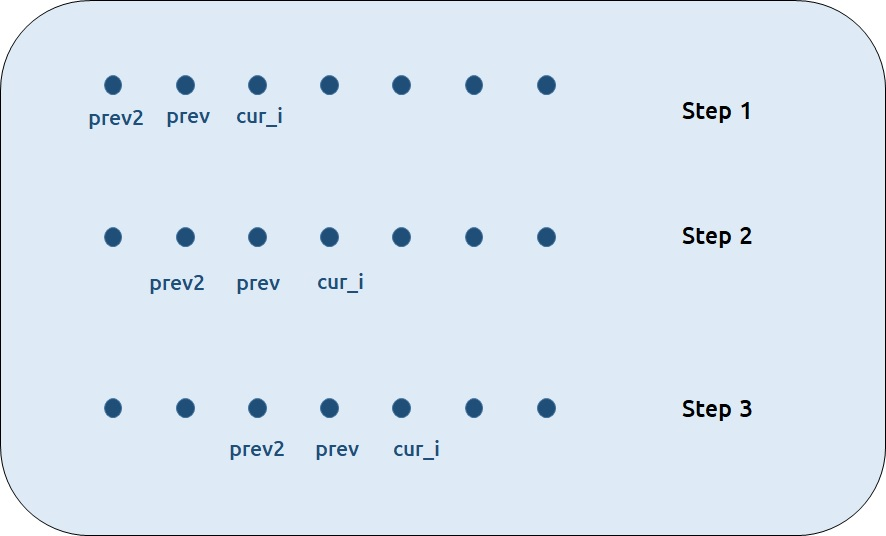• Each iteration’s cur_i and prev becomes the next iteration’s prev and prev2 respectively.
• Therefore after calculating cur_i, if we update prev and prev2 according to the next step, we will always get the answer.
• After the iterative loop has ended we can simply return prev as our answer.

Code:

## C++ Code

``````#include <bits/stdc++.h>

using namespace std;

int main() {

vector<int> height{30,10,60,10,60,50};
int n=height.size();
int prev=0;
int prev2=0;
for(int i=1;i<n;i++){

int jumpTwo = INT_MAX;
int jumpOne= prev + abs(height[i]-height[i-1]);
if(i>1)
jumpTwo = prev2 + abs(height[i]-height[i-2]);

int cur_i=min(jumpOne, jumpTwo);
prev2=prev;
prev=cur_i;

}
cout<<prev;
}
``````

Output: 40

Time Complexity: O(N)

Reason: We are running a simple iterative loop

Space Complexity: O(1)

Reason: We are not using any extra space.

## Java Code

``````import java.util.*;
class TUF{
public static void main(String args[]) {

int height[]={30,10,60,10,60,50};
int n=height.length;
int prev=0;
int prev2=0;
for(int i=1;i<n;i++){

int jumpTwo = Integer.MAX_VALUE;
int jumpOne= prev + Math.abs(height[i]-height[i-1]);
if(i>1)
jumpTwo = prev2 + Math.abs(height[i]-height[i-2]);

int cur_i=Math.min(jumpOne, jumpTwo);
prev2=prev;
prev=cur_i;

}
System.out.println(prev);
}
}``````

Output: 40

Time Complexity: O(N)

Reason: We are running a simple iterative loop

Space Complexity: O(1)

Reason: We are not using any extra space.

Special thanks to Anshuman Sharma for contributing to this article on takeUforward. If you also wish to share your knowledge with the takeUforward fam, please check out this article